Скачать презентацию Loop Iteration structures Booleans Iteration just means

a59efdf64b9c82bd3564770ce1ca8124.ppt

• Количество слайдов: 41Loop/Iteration structures & Booleans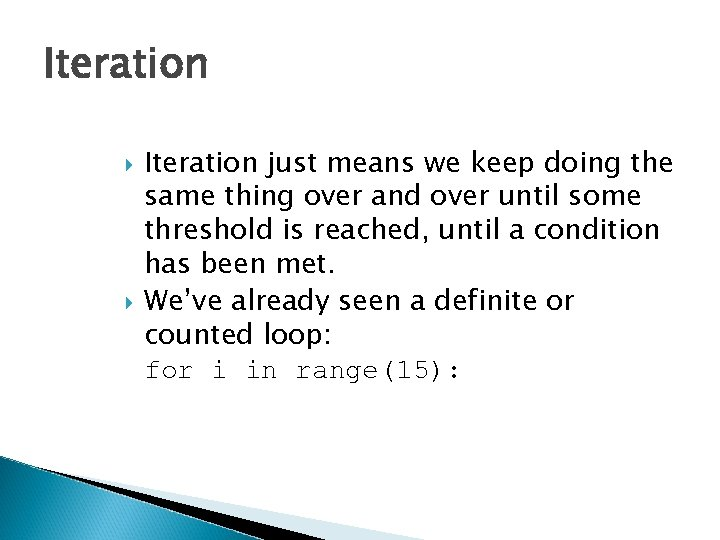Iteration just means we keep doing the same thing over and over until some threshold is reached, until a condition has been met. We’ve already seen a definite or counted loop: for i in range(15):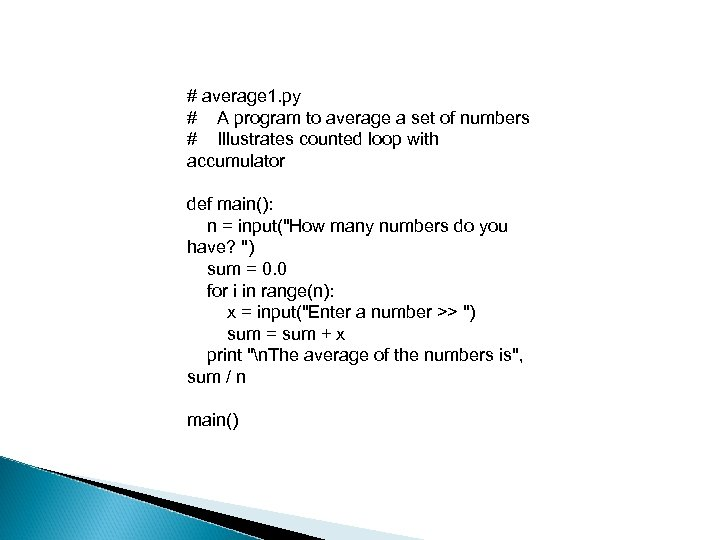# average 1. py # A program to average a set of numbers # Illustrates counted loop with accumulator def main(): n = input("How many numbers do you have? ") sum = 0. 0 for i in range(n): x = input("Enter a number >> ") sum = sum + x print "n. The average of the numbers is", sum / n main()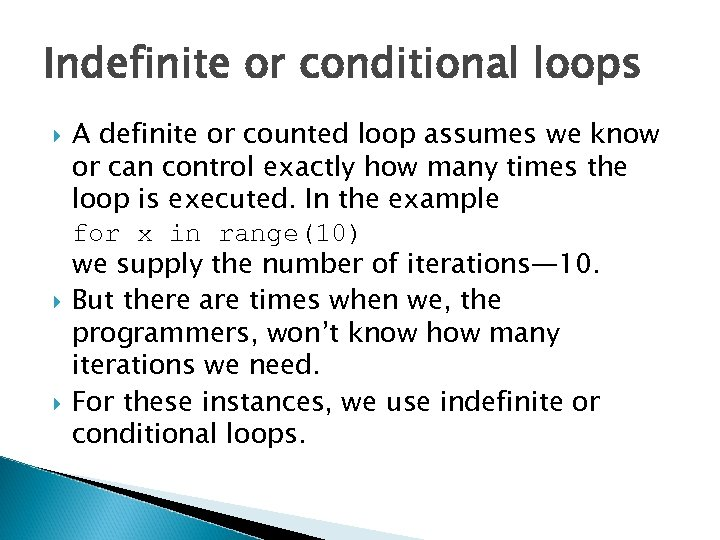Indefinite or conditional loops A definite or counted loop assumes we know or can control exactly how many times the loop is executed. In the example for x in range(10) we supply the number of iterations— 10. But there are times when we, the programmers, won’t know how many iterations we need. For these instances, we use indefinite or conditional loops.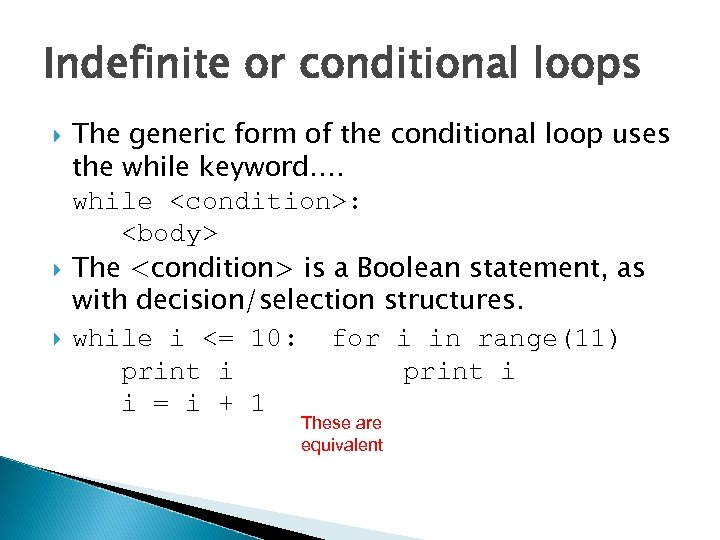Indefinite or conditional loops The generic form of the conditional loop uses the while keyword…. while : The is a Boolean statement, as with decision/selection structures. while i <= 10: for i in range(11) print i i = i + 1 These are equivalent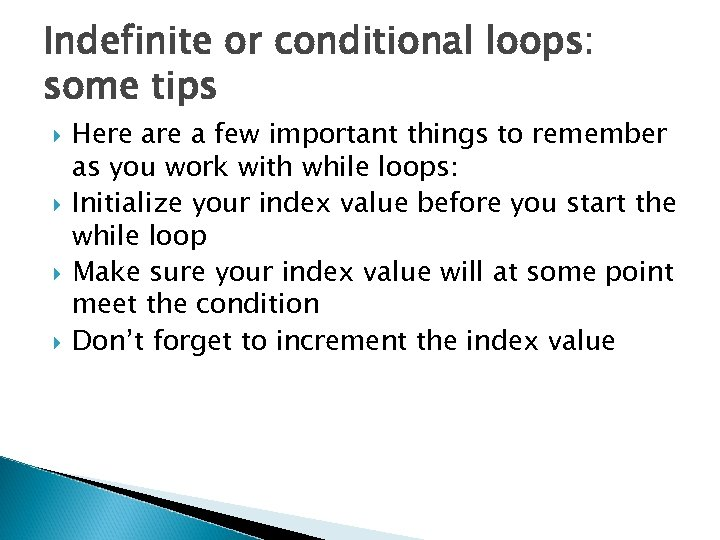Indefinite or conditional loops: some tips Here a few important things to remember as you work with while loops: Initialize your index value before you start the while loop Make sure your index value will at some point meet the condition Don’t forget to increment the index value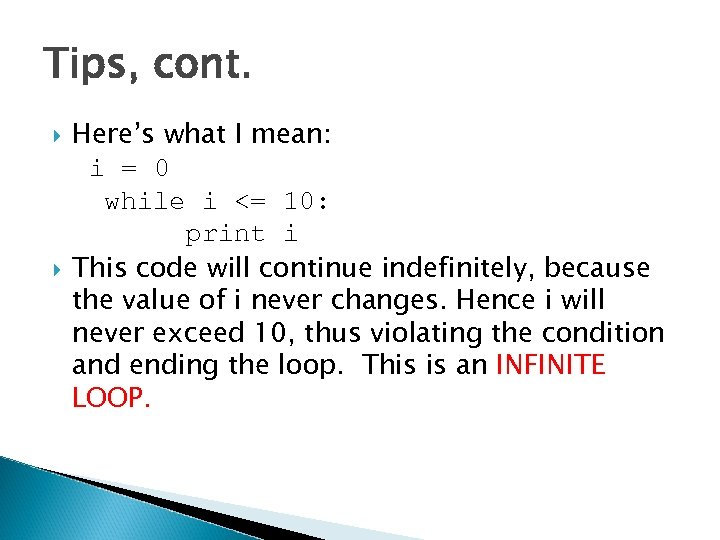Tips, cont. Here’s what I mean: i = 0 while i <= 10: print i This code will continue indefinitely, because the value of i never changes. Hence i will never exceed 10, thus violating the condition and ending the loop. This is an INFINITE LOOP.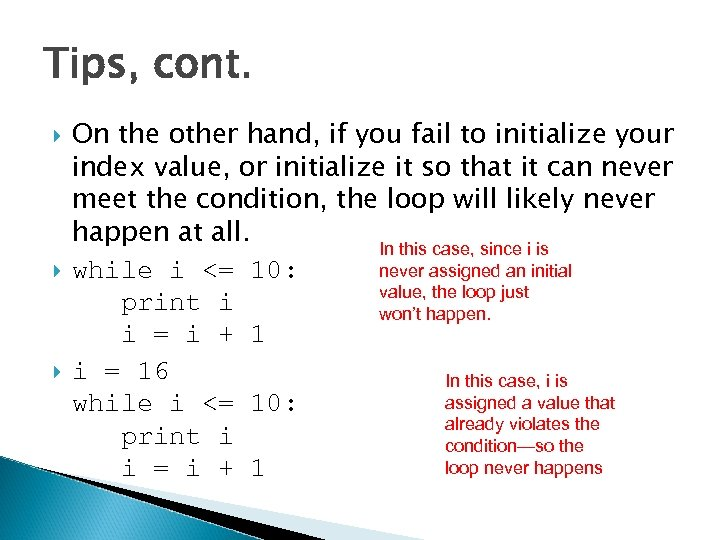Tips, cont. On the other hand, if you fail to initialize your index value, or initialize it so that it can never meet the condition, the loop will likely never happen at all. In this case, since i is never assigned an initial while i <= 10: value, the loop just print i won’t happen. i = i + 1 i = 16 In this case, i is assigned a value that while i <= 10: already violates the print i condition—so the loop never happens i = i + 1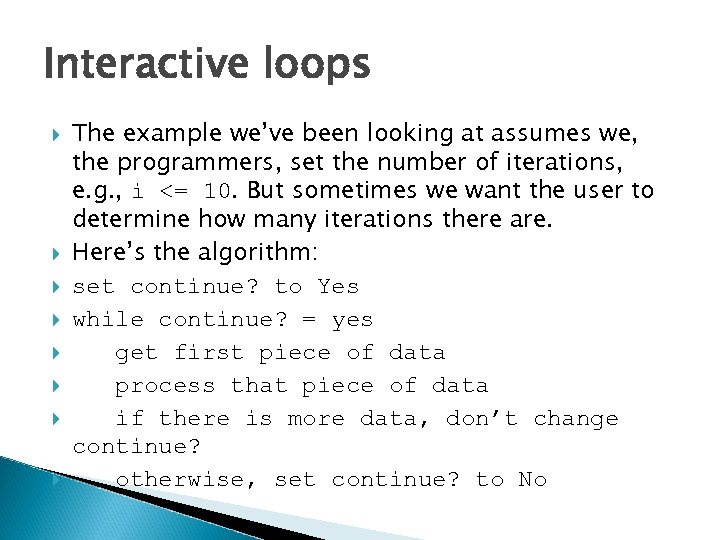Interactive loops The example we’ve been looking at assumes we, the programmers, set the number of iterations, e. g. , i <= 10. But sometimes we want the user to determine how many iterations there are. Here’s the algorithm: set continue? to Yes while continue? = yes get first piece of data process that piece of data if there is more data, don’t change continue? otherwise, set continue? to No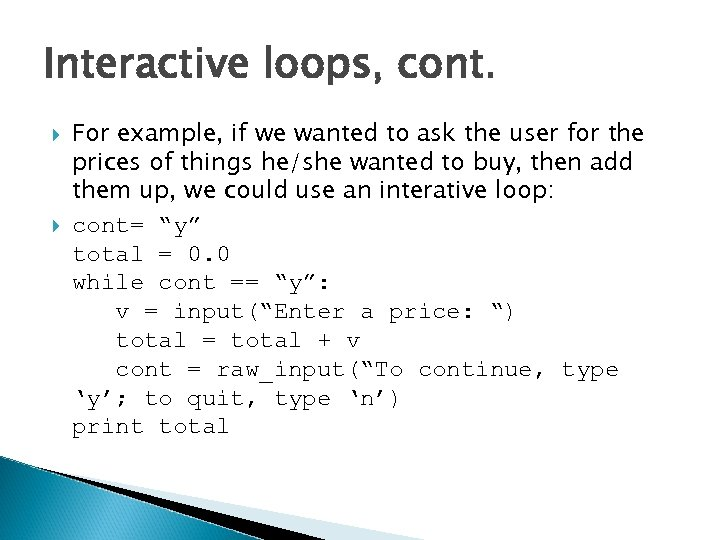Interactive loops, cont. For example, if we wanted to ask the user for the prices of things he/she wanted to buy, then add them up, we could use an interative loop: cont= “y” total = 0. 0 while cont == “y”: v = input(“Enter a price: “) total = total + v cont = raw_input(“To continue, type ‘y’; to quit, type ‘n’) print total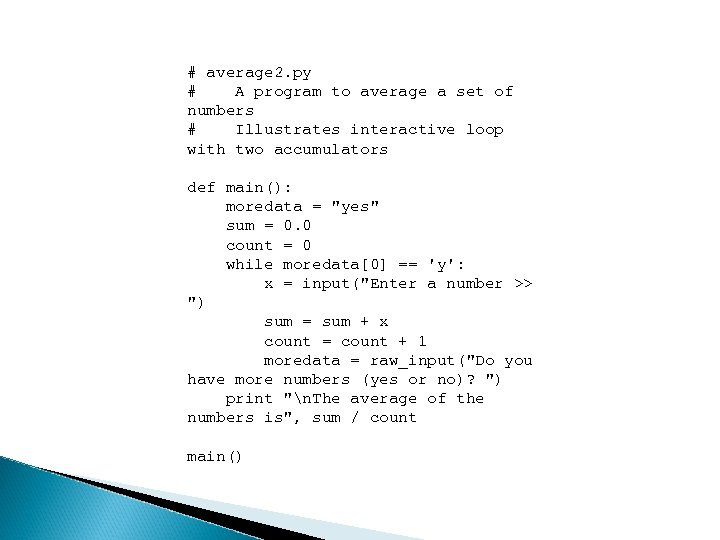# average 2. py # A program to average a set of numbers # Illustrates interactive loop with two accumulators def main(): moredata = "yes" sum = 0. 0 count = 0 while moredata == 'y': x = input("Enter a number >> ") sum = sum + x count = count + 1 moredata = raw_input("Do you have more numbers (yes or no)? ") print "n. The average of the numbers is", sum / count main()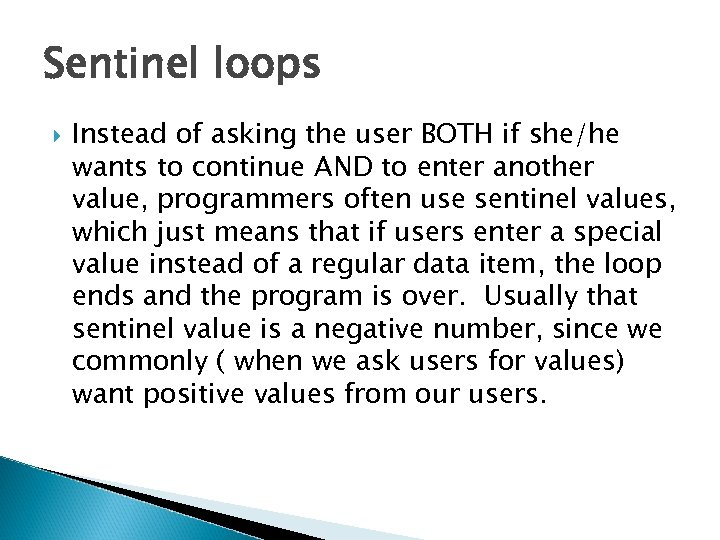Sentinel loops Instead of asking the user BOTH if she/he wants to continue AND to enter another value, programmers often use sentinel values, which just means that if users enter a special value instead of a regular data item, the loop ends and the program is over. Usually that sentinel value is a negative number, since we commonly ( when we ask users for values) want positive values from our users.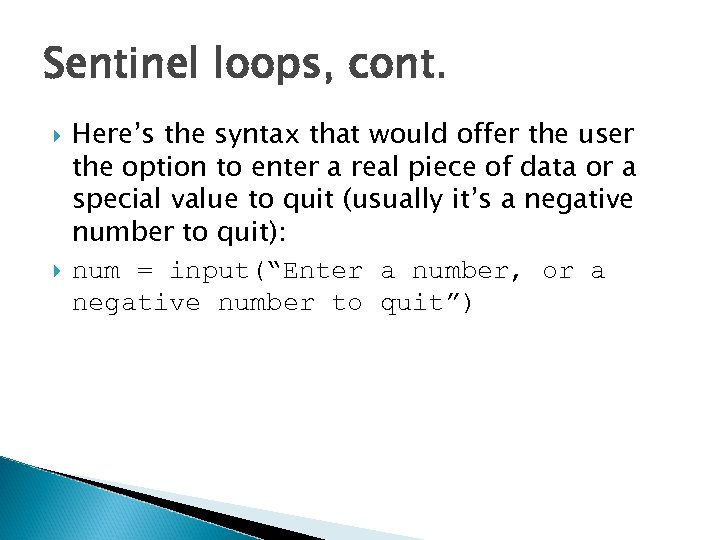Sentinel loops, cont. Here’s the syntax that would offer the user the option to enter a real piece of data or a special value to quit (usually it’s a negative number to quit): num = input(“Enter a number, or a negative number to quit”)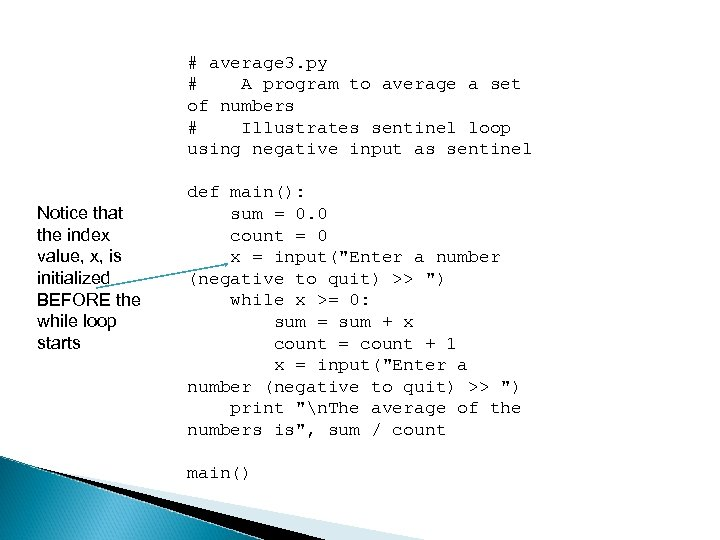# average 3. py # A program to average a set of numbers # Illustrates sentinel loop using negative input as sentinel Notice that the index value, x, is initialized BEFORE the while loop starts def main(): sum = 0. 0 count = 0 x = input("Enter a number (negative to quit) >> ") while x >= 0: sum = sum + x count = count + 1 x = input("Enter a number (negative to quit) >> ") print "n. The average of the numbers is", sum / count main()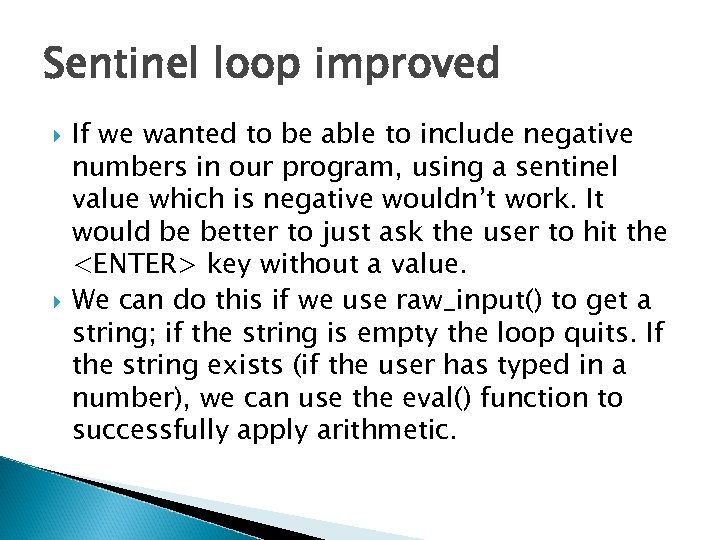Sentinel loop improved If we wanted to be able to include negative numbers in our program, using a sentinel value which is negative wouldn’t work. It would be better to just ask the user to hit the key without a value. We can do this if we use raw_input() to get a string; if the string is empty the loop quits. If the string exists (if the user has typed in a number), we can use the eval() function to successfully apply arithmetic.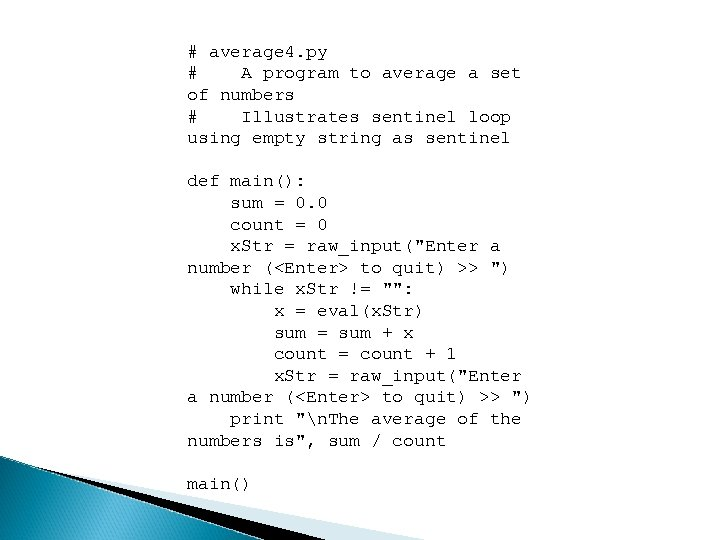# average 4. py # A program to average a set of numbers # Illustrates sentinel loop using empty string as sentinel def main(): sum = 0. 0 count = 0 x. Str = raw_input("Enter a number ( to quit) >> ") while x. Str != "": x = eval(x. Str) sum = sum + x count = count + 1 x. Str = raw_input("Enter a number ( to quit) >> ") print "n. The average of the numbers is", sum / count main()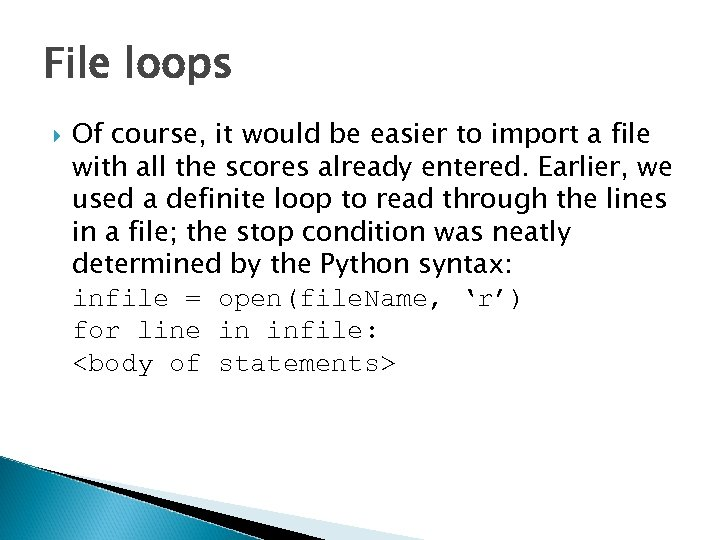File loops Of course, it would be easier to import a file with all the scores already entered. Earlier, we used a definite loop to read through the lines in a file; the stop condition was neatly determined by the Python syntax: infile = open(file. Name, ‘r’) for line in infile: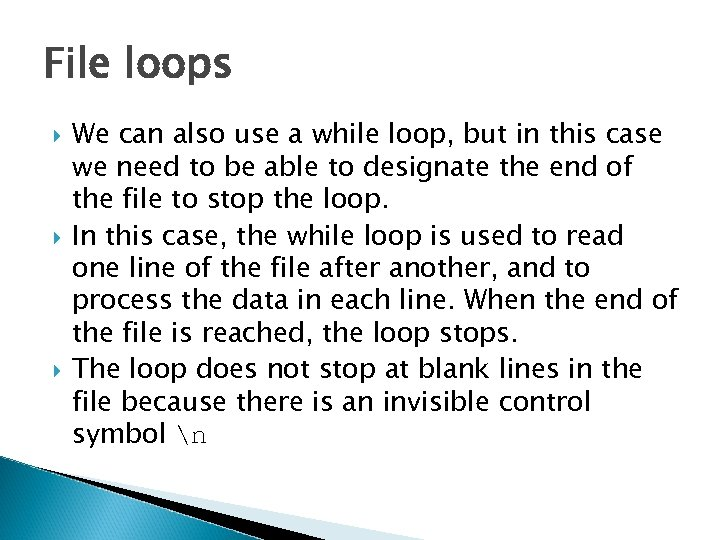File loops We can also use a while loop, but in this case we need to be able to designate the end of the file to stop the loop. In this case, the while loop is used to read one line of the file after another, and to process the data in each line. When the end of the file is reached, the loop stops. The loop does not stop at blank lines in the file because there is an invisible control symbol n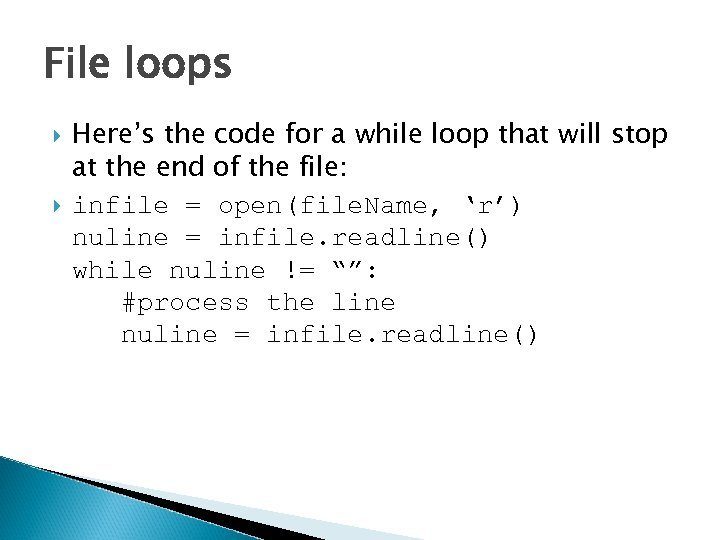File loops Here’s the code for a while loop that will stop at the end of the file: infile = open(file. Name, ‘r’) nuline = infile. readline() while nuline != “”: #process the line nuline = infile. readline()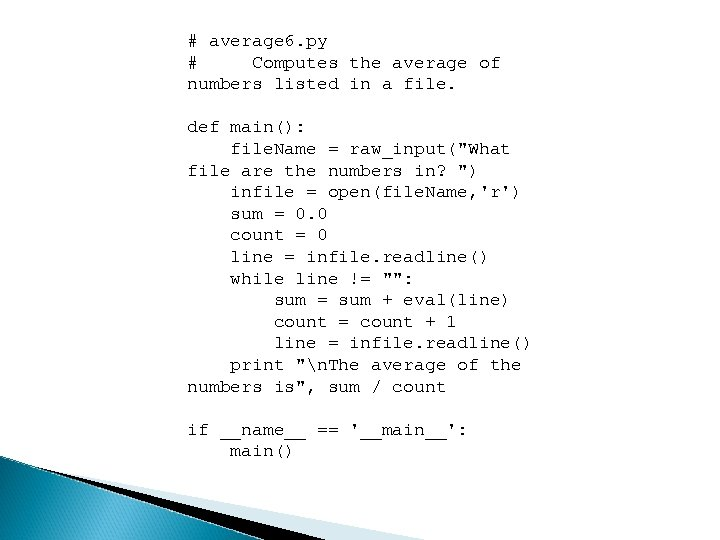# average 6. py # Computes the average of numbers listed in a file. def main(): file. Name = raw_input("What file are the numbers in? ") infile = open(file. Name, 'r') sum = 0. 0 count = 0 line = infile. readline() while line != "": sum = sum + eval(line) count = count + 1 line = infile. readline() print "n. The average of the numbers is", sum / count if __name__ == '__main__': main()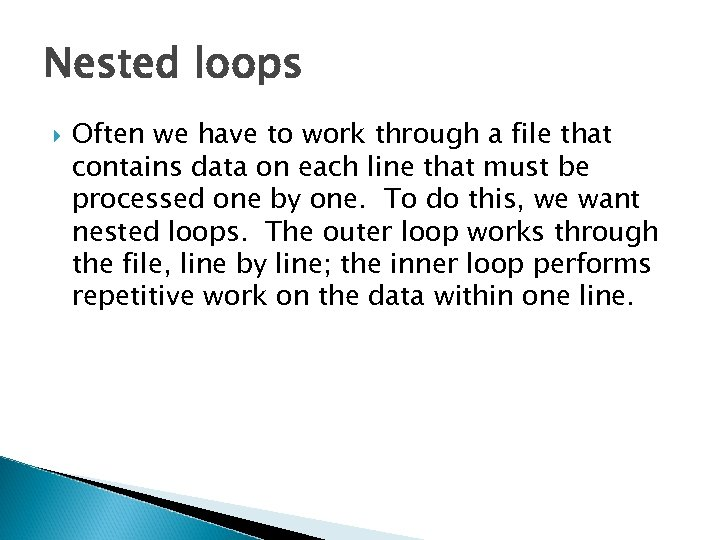Nested loops Often we have to work through a file that contains data on each line that must be processed one by one. To do this, we want nested loops. The outer loop works through the file, line by line; the inner loop performs repetitive work on the data within one line.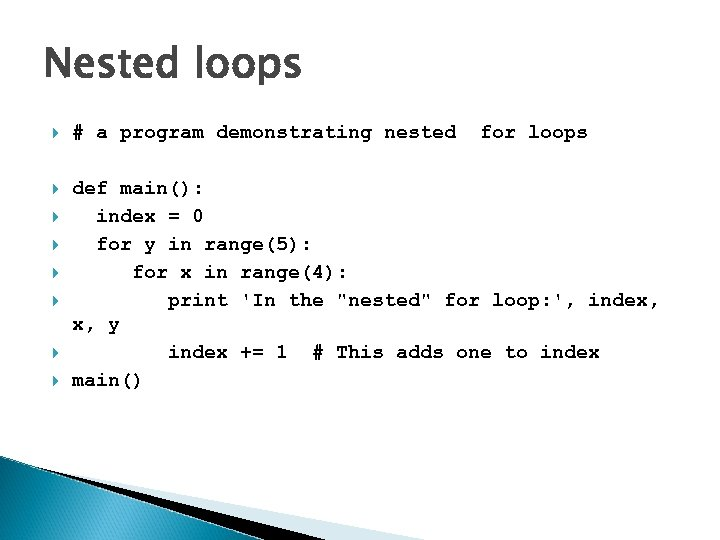Nested loops # a program demonstrating nested def main(): index = 0 for y in range(5): for x in range(4): print 'In the "nested" for loop: ', index, x, y index += 1 # This adds one to index main() for loops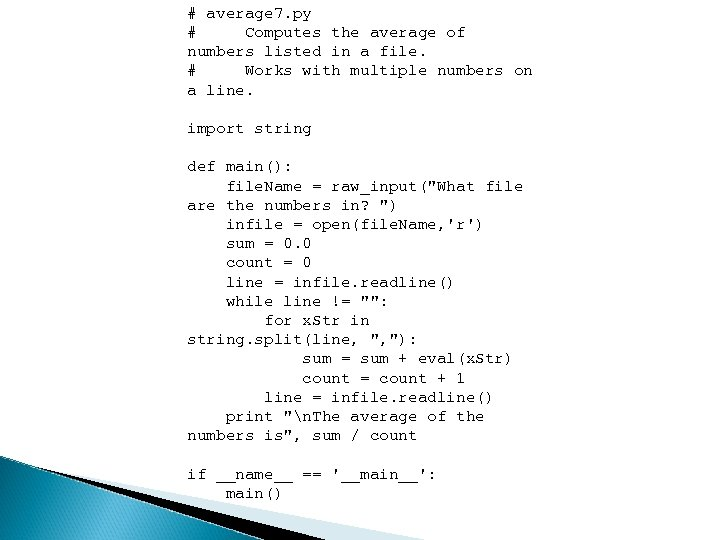# average 7. py # Computes the average of numbers listed in a file. # Works with multiple numbers on a line. import string def main(): file. Name = raw_input("What file are the numbers in? ") infile = open(file. Name, 'r') sum = 0. 0 count = 0 line = infile. readline() while line != "": for x. Str in string. split(line, ", "): sum = sum + eval(x. Str) count = count + 1 line = infile. readline() print "n. The average of the numbers is", sum / count if __name__ == '__main__': main()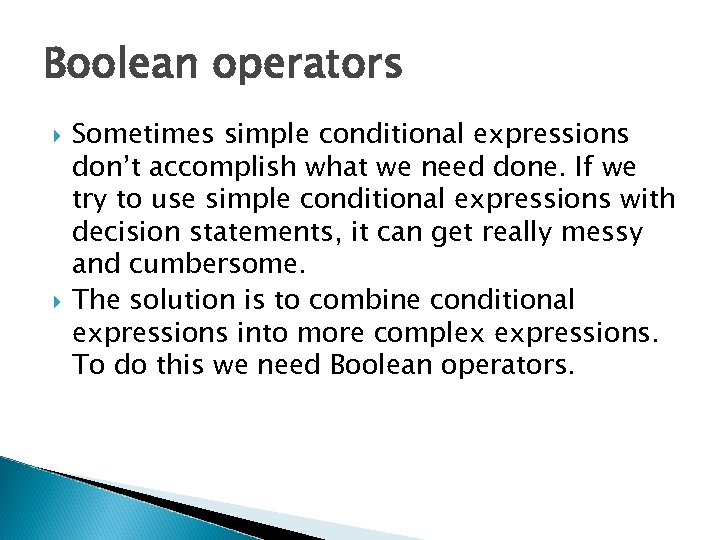Boolean operators Sometimes simple conditional expressions don’t accomplish what we need done. If we try to use simple conditional expressions with decision statements, it can get really messy and cumbersome. The solution is to combine conditional expressions into more complex expressions. To do this we need Boolean operators.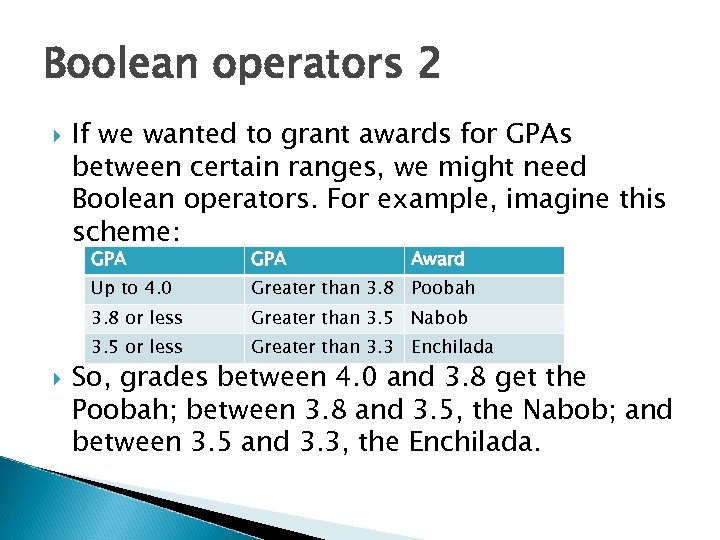Boolean operators 2 If we wanted to grant awards for GPAs between certain ranges, we might need Boolean operators. For example, imagine this scheme: GPA Up to 4. 0 Greater than 3. 8 Poobah 3. 8 or less Greater than 3. 5 Nabob 3. 5 or less GPA Award Greater than 3. 3 Enchilada So, grades between 4. 0 and 3. 8 get the Poobah; between 3. 8 and 3. 5, the Nabob; and between 3. 5 and 3. 3, the Enchilada.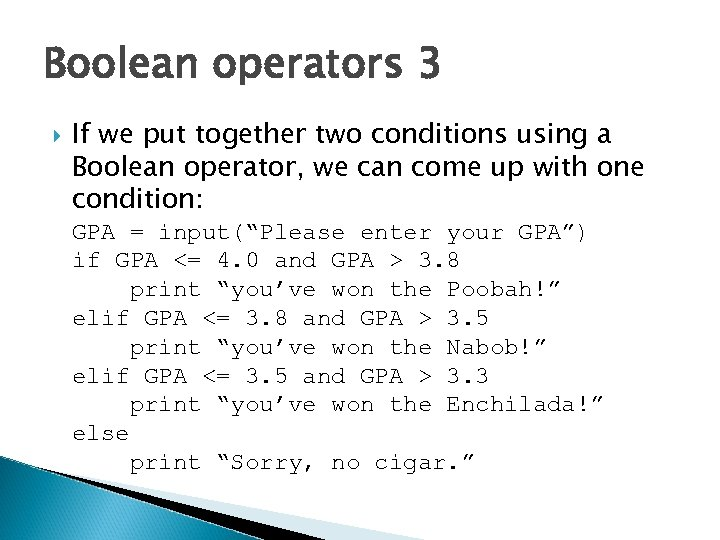Boolean operators 3 If we put together two conditions using a Boolean operator, we can come up with one condition: GPA = input(“Please enter your GPA”) if GPA <= 4. 0 and GPA > 3. 8 print “you’ve won the Poobah!” elif GPA <= 3. 8 and GPA > 3. 5 print “you’ve won the Nabob!” elif GPA <= 3. 5 and GPA > 3. 3 print “you’ve won the Enchilada!” else print “Sorry, no cigar. ”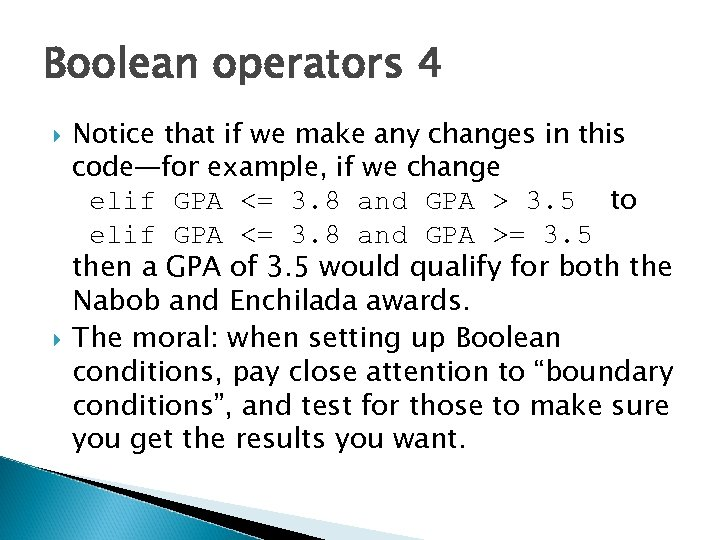Boolean operators 4 Notice that if we make any changes in this code—for example, if we change elif GPA <= 3. 8 and GPA > 3. 5 to elif GPA <= 3. 8 and GPA >= 3. 5 then a GPA of 3. 5 would qualify for both the Nabob and Enchilada awards. The moral: when setting up Boolean conditions, pay close attention to “boundary conditions”, and test for those to make sure you get the results you want.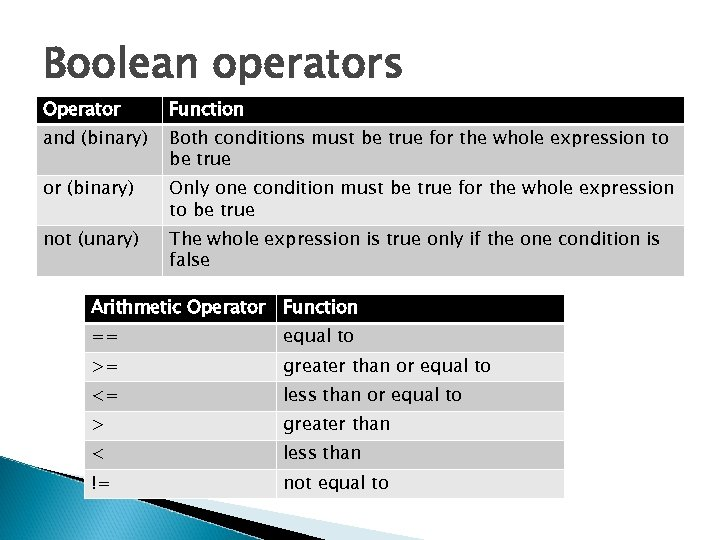Boolean operators Operator Function and (binary) Both conditions must be true for the whole expression to be true or (binary) Only one condition must be true for the whole expression to be true not (unary) The whole expression is true only if the one condition is false Arithmetic Operator Function == equal to >= greater than or equal to <= less than or equal to > greater than < less than != not equal to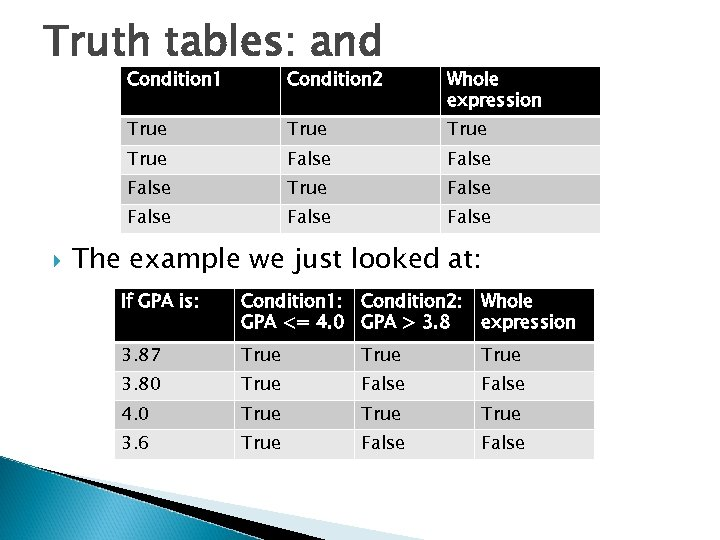Truth tables: and Condition 1 Whole expression True False True False Condition 2 False The example we just looked at: If GPA is: Condition 1: Condition 2: Whole GPA <= 4. 0 GPA > 3. 8 expression 3. 87 True 3. 80 True False 4. 0 True 3. 6 True False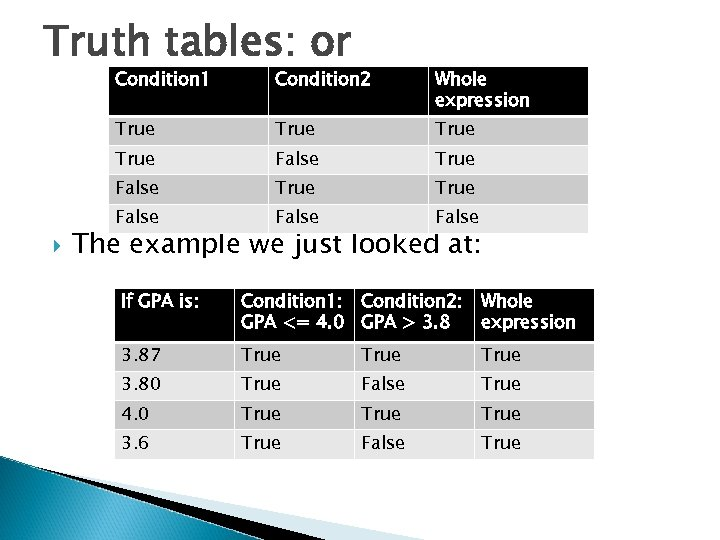Truth tables: or Condition 1 Whole expression True True False Condition 2 False The example we just looked at: If GPA is: Condition 1: Condition 2: Whole GPA <= 4. 0 GPA > 3. 8 expression 3. 87 True 3. 80 True False True 4. 0 True 3. 6 True False True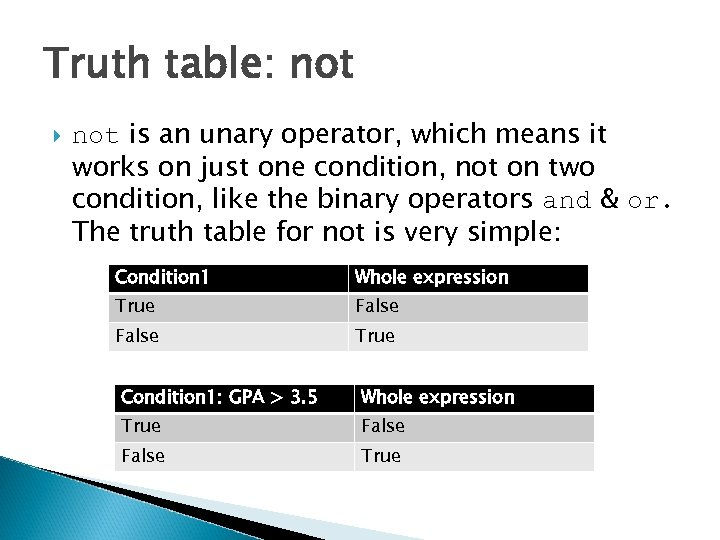Truth table: not is an unary operator, which means it works on just one condition, not on two condition, like the binary operators and & or. The truth table for not is very simple: Condition 1 Whole expression True False True Condition 1: GPA > 3. 5 Whole expression True False True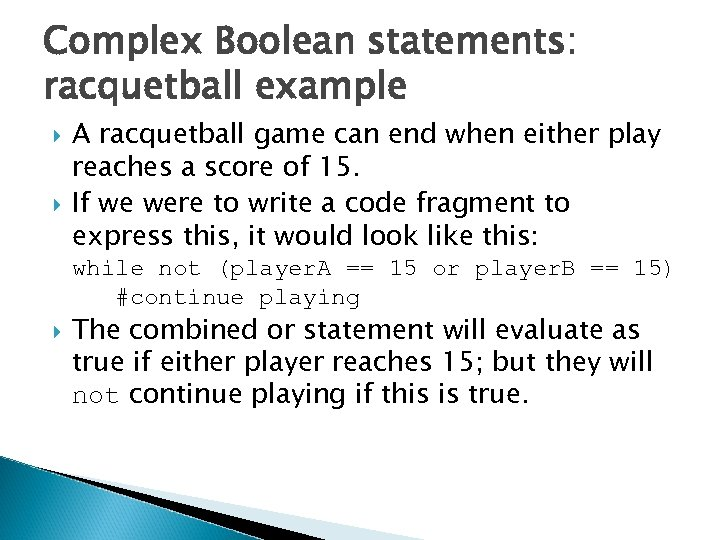Complex Boolean statements: racquetball example A racquetball game can end when either play reaches a score of 15. If we were to write a code fragment to express this, it would look like this: while not (player. A == 15 or player. B == 15) #continue playing The combined or statement will evaluate as true if either player reaches 15; but they will not continue playing if this is true.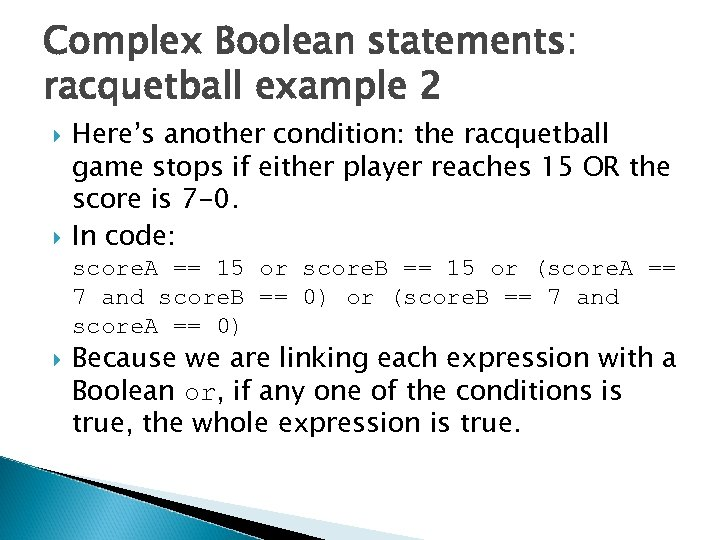Complex Boolean statements: racquetball example 2 Here’s another condition: the racquetball game stops if either player reaches 15 OR the score is 7 -0. In code: score. A == 15 or score. B == 15 or (score. A == 7 and score. B == 0) or (score. B == 7 and score. A == 0) Because we are linking each expression with a Boolean or, if any one of the conditions is true, the whole expression is true.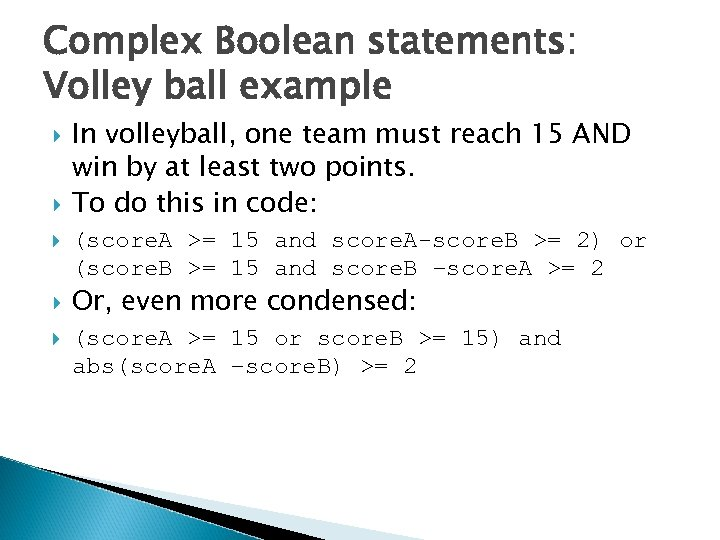Complex Boolean statements: Volley ball example In volleyball, one team must reach 15 AND win by at least two points. To do this in code: (score. A >= 15 and score. A-score. B >= 2) or (score. B >= 15 and score. B –score. A >= 2 Or, even more condensed: (score. A >= 15 or score. B >= 15) and abs(score. A –score. B) >= 2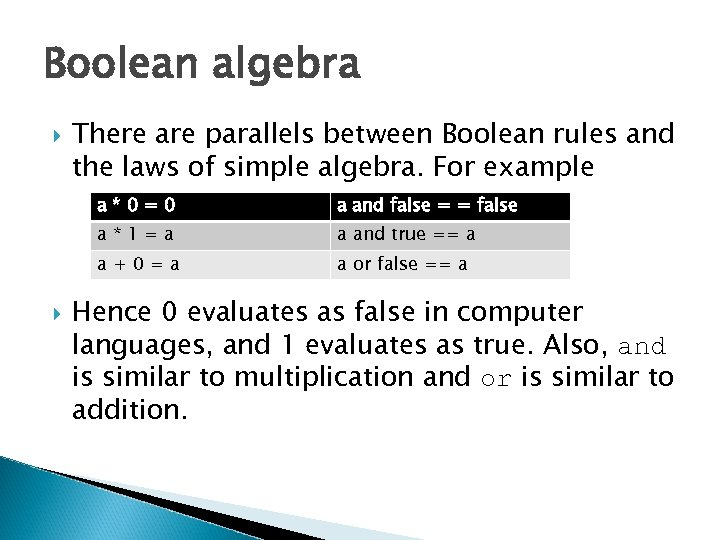Boolean algebra There are parallels between Boolean rules and the laws of simple algebra. For example a*0=0 a*1=a a and true == a a+0=a a and false = = false a or false == a Hence 0 evaluates as false in computer languages, and 1 evaluates as true. Also, and is similar to multiplication and or is similar to addition.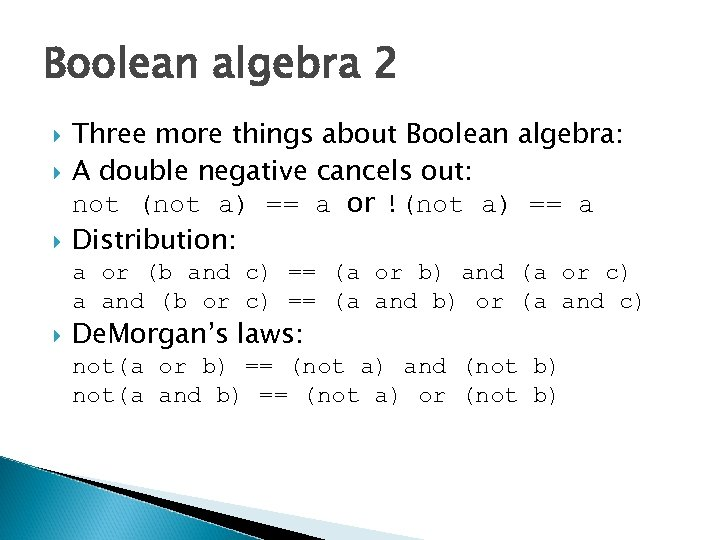Boolean algebra 2 Three more things about Boolean algebra: A double negative cancels out: not (not a) == a or !(not a) == a Distribution: a or (b and c) == (a or b) and (a or c) a and (b or c) == (a and b) or (a and c) De. Morgan’s laws: not(a or b) == (not a) and (not b) not(a and b) == (not a) or (not b)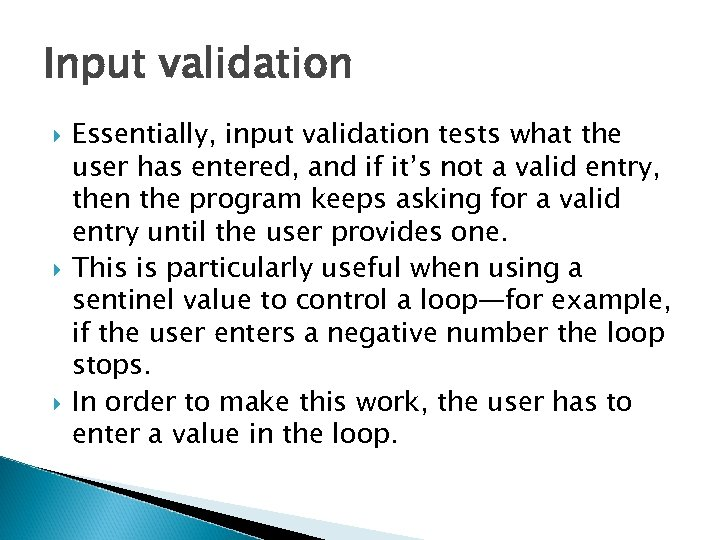Input validation Essentially, input validation tests what the user has entered, and if it’s not a valid entry, then the program keeps asking for a valid entry until the user provides one. This is particularly useful when using a sentinel value to control a loop—for example, if the user enters a negative number the loop stops. In order to make this work, the user has to enter a value in the loop.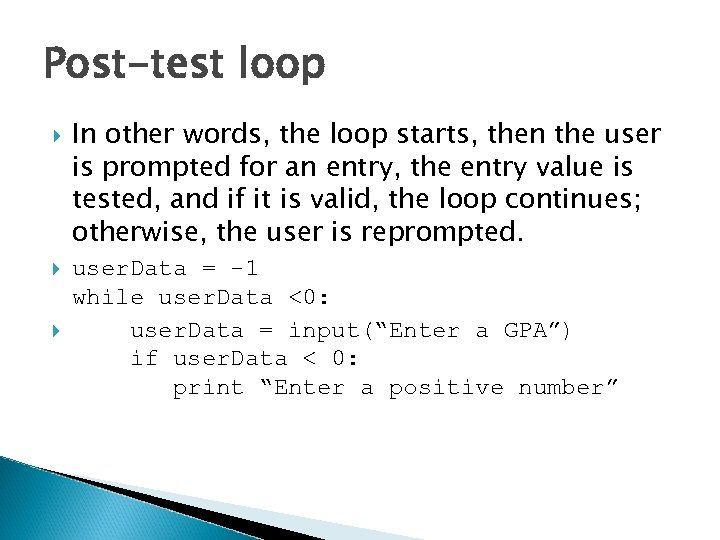Post-test loop In other words, the loop starts, then the user is prompted for an entry, the entry value is tested, and if it is valid, the loop continues; otherwise, the user is reprompted. user. Data = -1 while user. Data <0: user. Data = input(“Enter a GPA”) if user. Data < 0: print “Enter a positive number”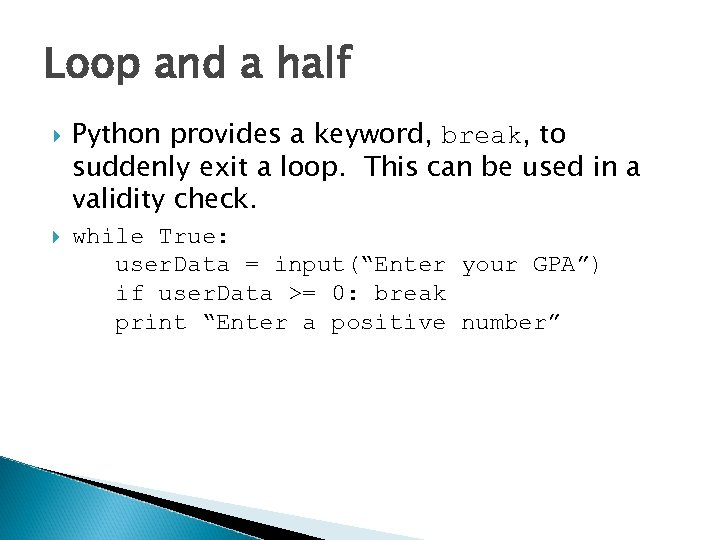Loop and a half Python provides a keyword, break, to suddenly exit a loop. This can be used in a validity check. while True: user. Data = input(“Enter your GPA”) if user. Data >= 0: break print “Enter a positive number”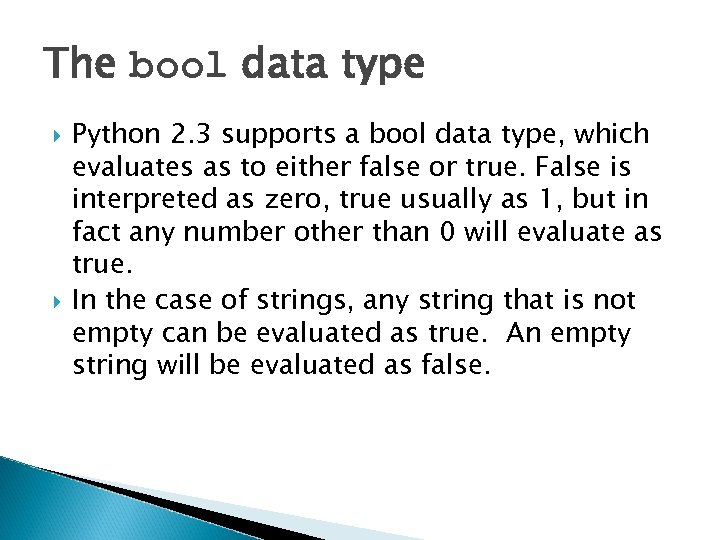The bool data type Python 2. 3 supports a bool data type, which evaluates as to either false or true. False is interpreted as zero, true usually as 1, but in fact any number other than 0 will evaluate as true. In the case of strings, any string that is not empty can be evaluated as true. An empty string will be evaluated as false.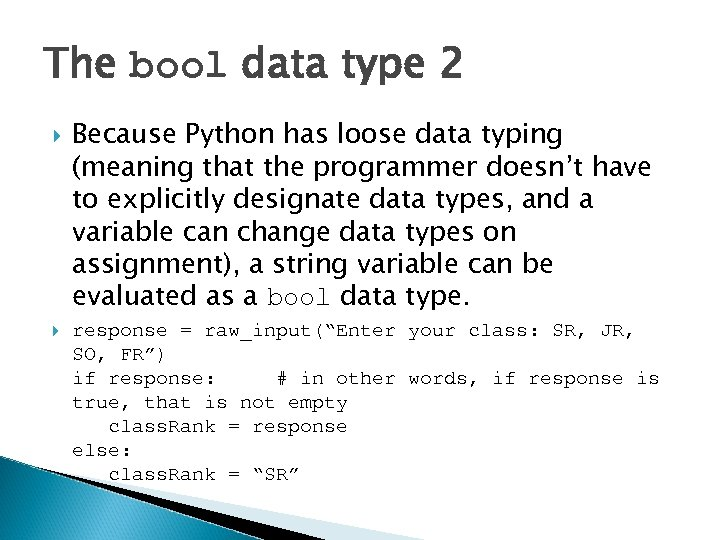The bool data type 2 Because Python has loose data typing (meaning that the programmer doesn’t have to explicitly designate data types, and a variable can change data types on assignment), a string variable can be evaluated as a bool data type. response = raw_input(“Enter your class: SR, JR, SO, FR”) if response: # in other words, if response is true, that is not empty class. Rank = response else: class. Rank = “SR”